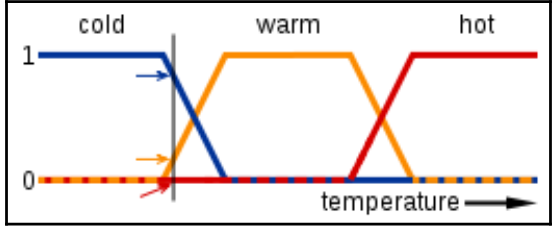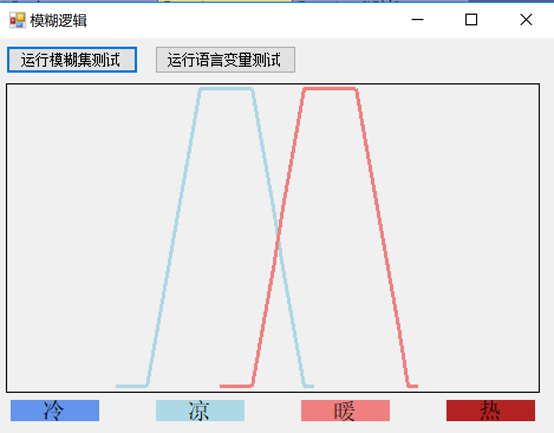# 基于C#的机器学习--模糊逻辑-穿越障碍# 模糊逻辑-穿越障碍"In this image, the meanings of the expressions cold, warm, and hot are represented by functions mapping a temperature scale. A point on that scale has three "truth values"-one for each of the three functions. The vertical line in the image represents a particular temperature that the three arrows (truth values) gauge. Since the red arrow points to zero,this temperature may be interpreted as "not hot". The orange arrow (pointing at 0.2) may describe it as "slightly warm" and the blue arrow (pointing at 0.8) "fairly cold"."

1. 模糊逻辑

2. 避障与识别

3. AGV

## 模糊逻辑`　　　　#region 创建两个模糊集来表示凉和暖的温度#region 凉TrapezoidalFunction function1 = new TrapezoidalFunction(13, 18, 23, 28);FuzzySet fsCool = new FuzzySet("凉", function1);double[,] coolValues = new double[20, 2];for (int i = 10; i < 30; i  ){ coolValues[i - 10, 0] = i; coolValues[i - 10, 1] = fsCool.GetMembership(i);}chart?.UpdateDataSeries("凉", coolValues);#endregion 凉#region 暖TrapezoidalFunction function2 = new TrapezoidalFunction(23, 28, 33, 38);FuzzySet fsWarm = new FuzzySet("暖", function2);double[,] warmValues = new double[20, 2];for (int i = 20; i < 40; i  ){ warmValues[i - 20, 0] = i; warmValues[i - 20, 1] = fsWarm.GetMembership(i);}chart?.UpdateDataSeries("暖", warmValues);#endregion 暖 　　　　#endregion 创建两个模糊集来表示凉和暖的温度`

`　　　　　　　LinguisticVariable lvTemperature = new LinguisticVariable("温度", 0, 80);TrapezoidalFunction function1 = new TrapezoidalFunction(10, 15, TrapezoidalFunction.EdgeType.Right);FuzzySet fsCold = new FuzzySet("冷", function1);lvTemperature.AddLabel(fsCold);TrapezoidalFunction function2 = new TrapezoidalFunction(10, 15, 20, 25);FuzzySet fsCool = new FuzzySet("凉", function2);lvTemperature.AddLabel(fsCool);TrapezoidalFunction function3 = new TrapezoidalFunction(20, 25, 30, 35);FuzzySet fsWarm = new FuzzySet("暖", function3);lvTemperature.AddLabel(fsWarm);TrapezoidalFunction function4 = new TrapezoidalFunction(30, 35, TrapezoidalFunction.EdgeType.Left);FuzzySet fsHot = new FuzzySet("热", function4);lvTemperature.AddLabel(fsHot);double[][,] chartValues = new double[,];for (int i = 0; i < 4; i  ) chartValues[i] = new double[160, 2];`

`  #region 语言变量的形状——它的标签从开始到结束的形状int j = 0;for (float x = 0; x < 80; x  = 0.5f, j  ){ double y1 = lvTemperature.GetLabelMembership("冷", x); double y2 = lvTemperature.GetLabelMembe`